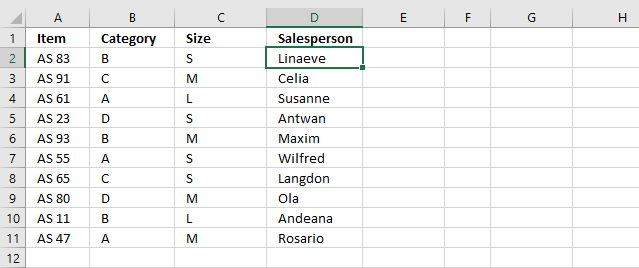Author: Oscar Cronquist Article last updated on October 19, 2020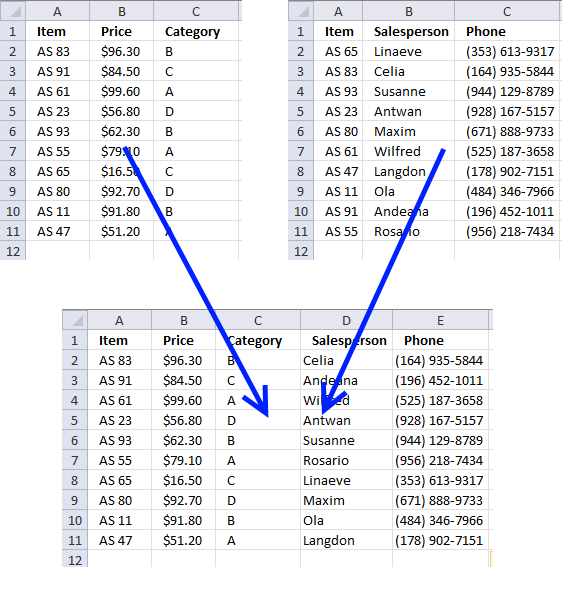This article demonstrates techniques on how to merge or combine two data sets using a condition. The top left data set has three headers, Item, Price, and Category. The top right data set has three columns,  header names are Item, Salesperson, and Phone.

Both data sets contain column Item, this allows you to combine them using the column item as a condition. I will in this article use different Excel functions to merge these data sets. The two last examples show how to combine data sets if a cell contains a given value and how to match multiple columns in order to combine the data sets.

I have written a post about merging two single columns or ranges before: Merge two columns with possible blank cells. It demonstrates how to merge two different columns dynamically and that is it. The following examples merge data tables with a condition or criteria.

### Example 1 - Vlookup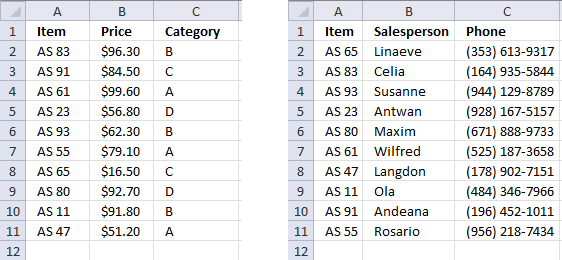The image above shows two tables on two different worksheets. They only share the same items, however, they are not in order making it harder and tedious to combine manually.

Let us use the Item value in the first table and search for it in the second table. The VLOOKUP function allows us to retrieve corresponding values.Here is the table in worksheet named sheet1 merged with the table located on worksheet named sheet2, see image above.

Formula in cell D2:

=VLOOKUP(\$A2, 'Ex 1 - Sheet2'!\$A\$2:\$C\$11, COLUMN(B1), FALSE)

Copy cell D2 and paste to E2. Then copy cell range D2:E2 and paste to cell range D3:E11.

#### Explaining formula in cell D2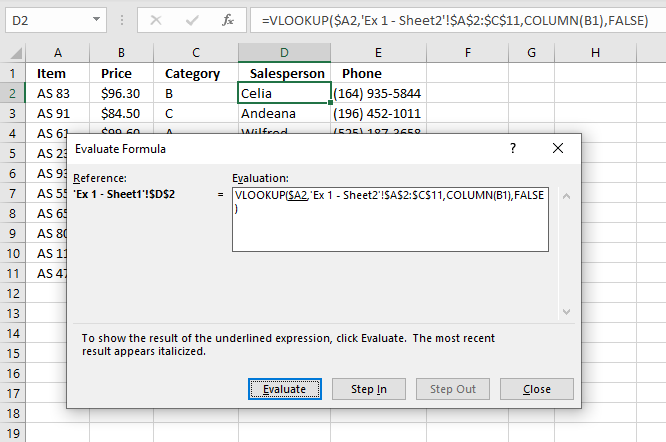I do recommend using the "Evaluate Formula" tool when debugging and examining formulas, it allows you to evaluate different parts of the formula individually. Going through the formula is as easy as press with left mouse button oning a button, Excel shows the result of each expression.

Here is how to start this tool. Select the cell you want to check out. Go to tab "Formulas" on the ribbon, press with left mouse button on the "Evaluate Formula" button. This opens a dialog box, see image above.

The "Evaluate Formula" dialog box shows the formula and the underlined expression is what is next to be evaluated, the most recent result from an evaluation is italicized.

Press with left mouse button on the "Evaluate" button to move to the next step in the formula calculation, keep press with left mouse button oning until you are satisfied or the final result appears. That result always match what the cell shows. Press with left mouse button on "Close" button to dismiss the dialog box.

##### Step 1 - VLOOKUP function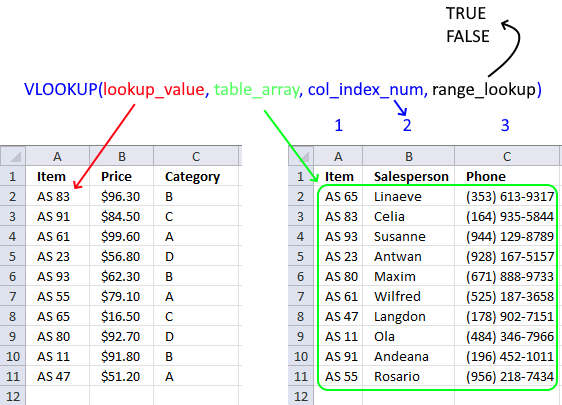The VLOOKUP function looks for a value in the leftmost column of a table and then returns a value in the same row from a column you specify.

The VLOOKUP function has four arguments: lookup_valuetable_arraycol_index_num ,range_lookup

VLOOKUP(lookup_valuetable_arraycol_index_num ,range_lookup)

The Item column is the leftmost column which is needed in order to use the VLOOKUP function, remember the Item column is shared by both data sets.

##### Step 2 - lookup_value argumentThe formula is entered in cell D2 and it uses the corresponding lookup value in cell \$A2 to find a match in the second data set located on worksheet Sheet2.

VLOOKUP(\$A2, 'Ex 1 - Sheet2'!\$A\$2:\$C\$11, COLUMN(B1), FALSE)

becomes

VLOOKUP("AS 83", 'Ex 1 - Sheet2'!\$A\$2:\$C\$11, COLUMN(B1), FALSE)

See the \$ sign? It makes column A absolute, meaning the column part of cell reference does not change when we copy cell D2 and paste to cell E2.

##### Step 3 - table_array argumentThe VLOOKUP function looks for the value in cell \$A2 in the leftmost column of cell range 'Ex 1 - Sheet2'!\$A\$2:\$C\$11.

It also returns a value from this cell range if there is a match based on the chosen column number in argument col_index_num. See the next step where I explain the col_index_num argument.

VLOOKUP("AS 82", 'Ex 1 - Sheet2'!\$A\$2:\$C\$11, COLUMN(B1), FALSE)

becomes

VLOOKUP("AS 82", {"AS 65", "Linaeve", "(353) 613-9317"; "AS 83", "Celia", "(164) 935-5844"; "AS 93", "Susanne", "(944) 129-8789"; "AS 23", "Antwan", "(928) 167-5157"; "AS 80", "Maxim", "(671) 888-9733"; "AS 61", "Wilfred", "(525) 187-3658"; "AS 47", "Langdon", "(178) 902-7151"; "AS 11", "Ola", "(484) 346-7966"; "AS 91", "Andeana", "(196) 452-1011"; "AS 55", "Rosario", "(956) 218-7434"}, COLUMN(B1), FALSE)

##### Step 4 - col_index_num argumentcol_index_num is a column number determining which column in cell 'Ex 1 - Sheet2'!\$A\$2:\$C\$11 we want to get values from.

I use COLUMN(B1) in this example, it returns 2 for formulas in column D and 3 for formulas in column E. Cell reference B1 is a relative cell reference meaning it changes when you copy the cell and pastes it to another cell.

VLOOKUP("AS 82", {"AS 65", "Linaeve", "(353) 613-9317"; "AS 83", "Celia", "(164) 935-5844"; "AS 93", "Susanne", "(944) 129-8789"; "AS 23", "Antwan", "(928) 167-5157"; "AS 80", "Maxim", "(671) 888-9733"; "AS 61", "Wilfred", "(525) 187-3658"; "AS 47", "Langdon", "(178) 902-7151"; "AS 11", "Ola", "(484) 346-7966"; "AS 91", "Andeana", "(196) 452-1011"; "AS 55", "Rosario", "(956) 218-7434"}, COLUMN(B1), FALSE)

becomes

VLOOKUP("AS 82", {"AS 65", "Linaeve", "(353) 613-9317"; "AS 83", "Celia", "(164) 935-5844"; "AS 93", "Susanne", "(944) 129-8789"; "AS 23", "Antwan", "(928) 167-5157"; "AS 80", "Maxim", "(671) 888-9733"; "AS 61", "Wilfred", "(525) 187-3658"; "AS 47", "Langdon", "(178) 902-7151"; "AS 11", "Ola", "(484) 346-7966"; "AS 91", "Andeana", "(196) 452-1011"; "AS 55", "Rosario", "(956) 218-7434"}, 2, FALSE)

##### Step 5 - range_lookup argumentThe range_lookup argument lets you chose if you want the VLOOKUP function to perform an aproximate or exact match. We want an exact match so we will use FALSE.

TRUE - Approximate match
FALSE - Exact match

An approximate match requires the values in the leftmost column in the table_array to be sorted in ascending order. This is not required when you use an exact match.

##### Step 6 - Return value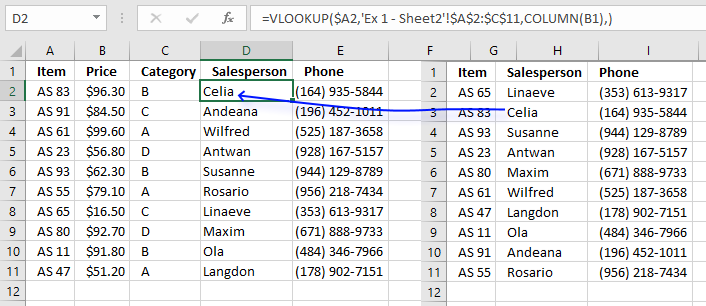The VLOOKUP function finds a match on row 3 and returns the value from the second column on worksheet Sheet2 which is "Celia" and returns that value to cell D2 on worksheet Sheet1.

### Example 2 - INDEX and MATCH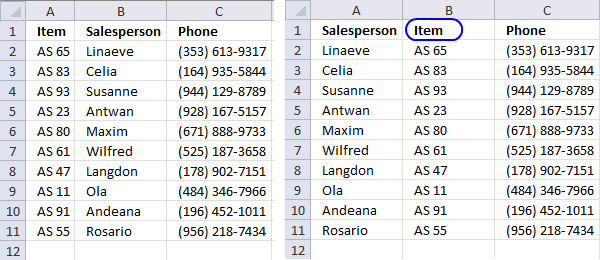The image above shows two lists on two different sheets. They share the same items, however, they are not sorted. The second list has "Items" in column B (blue circle).

You can't use the VLOOKUP function now unless you move the Items column to the leftmost column in the cell range.  But wait, you don't have to move the Items column, you can use a formula that combines two Excel functions.

If you combine the INDEX and MATCH function you can build a formula that is more versatile and as easy to use as the VLOOKUP function. This picture shows the merged list using a formula containing the INDEX and MATCH functions.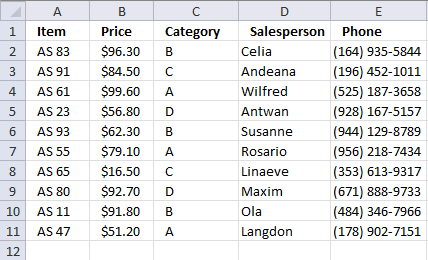Formula in cell D2:

=INDEX('Ex 2 - Sheet2'!\$A\$2:\$C\$11, MATCH('Ex 2 - Sheet1'!A2,'Ex 2 - Sheet2'!\$B\$2:\$B\$11, 0), 1)

Copy cell D2 to cell range D3:D11.

Formula in cell E2:

=INDEX('Ex 2 - Sheet2'!\$A\$2:\$C\$11, MATCH('Ex 2 - Sheet1'!A2,'Ex 2 - Sheet2'!\$B\$2:\$B\$11, 0), 3)

Copy cell E2 to cell range E3:E11.

The only difference between these two formulas is the column number that the INDEX function uses to determine which value to extract.

#### Explaining formula in cell D2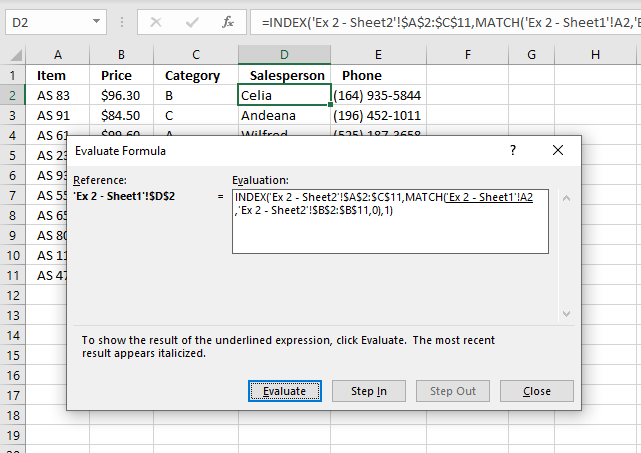The following link takes you to an explanation of how the "Evaluate Formula" tool works.

##### Step 1 - Find the relative position with the value in cell A2 in cell range 'Ex 2 - Sheet2'!\$B\$2:\$B\$11

The MATCH function returns a number representing the relative position of a given value (lookup_value argument) in a one-dimensional array or cell range (lookup_array argument).

MATCH(lookup_value, lookup_array, [match_type])

The match_type argument can be -1, 0, and 1. 0 (zero) is an exact match. Check out the other match_type arguments.

MATCH('Ex 2 - Sheet1'!A2,'Ex 2 - Sheet2'!\$B\$2:\$B\$11,0)

becomes

MATCH("As 83",{"AS 65";"AS 83";"AS 93";"AS 23";"AS 80";"AS 61";"AS 47";"AS 11";"AS 91";"AS 55"},0)

and returns 2. This means that the lookup_value is found in the second position in cell range 'Ex 2 - Sheet2'!\$B\$2:\$B\$11 which you can verify if you check out the array above.

##### Step 2 - Return a value of the cell at the intersection of a particular row and column

The INDEX function returns a value from a cell range or array based on a row and column number (both optional).

INDEX(array[row_num][column_num])

INDEX('Ex 2 - Sheet2'!\$A\$2:\$C\$11,MATCH('Ex 2 - Sheet1'!A2,'Ex 2 - Sheet2'!\$B\$2:\$B\$11,0),1)

becomes

INDEX('Ex 2 - Sheet2'!\$A\$2:\$C\$11,2,1)

The MATCH function returned 2 which we will use in the second argument row_num. The third argument is 1 because we want to get the value from the first column.

becomes

INDEX({"Linaeve","AS 65","(353) 613-9317"; "Celia","AS 83","(164) 935-5844"; "Susanne","AS 93","(944) 129-8789"; "Antwan","AS 23","(928) 167-5157"; "Maxim","AS 80","(671) 888-9733"; "Wilfred","AS 61","(525) 187-3658"; "Langdon","AS 47","(178) 902-7151"; "Ola","AS 11","(484) 346-7966"; "Andeana","AS 91","(196) 452-1011"; "Rosario","AS 55","(956) 218-7434"},2,1)

and returns value "Celia" from row 2 and column 1 in cell range 'Ex 2 - Sheet2'!\$A\$2:\$C\$11.

### Example 3 - Wild card search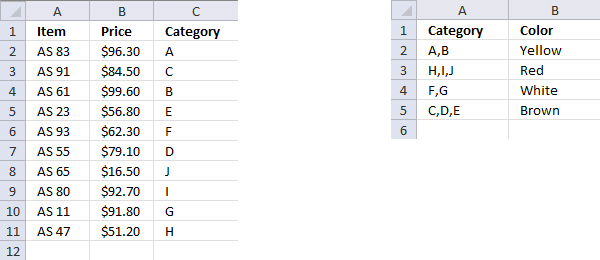Here we want to find the color for each category, the first data set contains category values in the third column. The second list contains multiple categories in column A and a corresponding color in column B.

This means that multiple categories may share the same color. This picture below shows the merged list.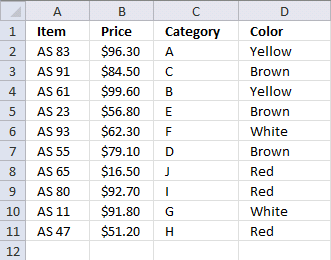Formula in cell D2:

=INDEX('Ex 3 - Sheet2'!\$B\$2:\$B\$5, MATCH("*"&C2&"*",'Ex 3 - Sheet2'!\$A\$2:\$A\$5, 0))

Copy cell D2 and paste it to cell range D3:D11.

You can use the VLOOKUP function in this example also, as long as you search in the leftmost column. I do, however,  prefer the INDEX and MATCH functions.

=VLOOKUP("*"&C2&"*", 'Ex 3 - Sheet2'!\$A\$2:\$B\$5, 2, FALSE)

You can use other characters, as well: Wildcard characters

### Explaining the array formula in cell D2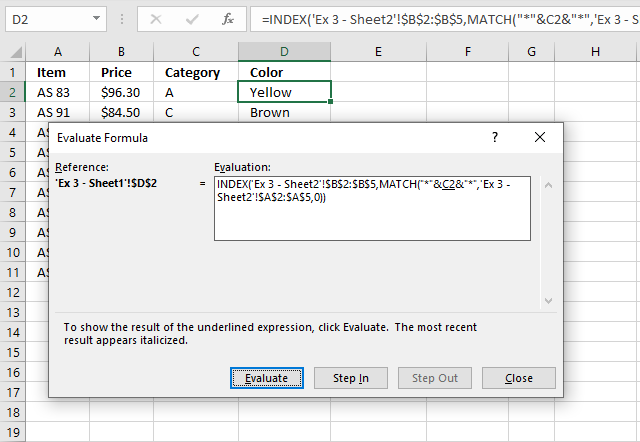The following link takes you to an explanation of how the "Evaluate Formula" tool works.

##### Step 1 - Search for a text string in an array of cell values and return relative position of first match in array

The MATCH function allows you to append asterisk characters before and after the lookup_value argument allowing you to search a cell to see if it contains a given value.

The MATCH function will match the entire cell if you don't append asterisks to the argument.

MATCH(lookup_value, lookup_array, [match_type])

MATCH("*"&C2&"*",'Ex 3 - Sheet2'!\$A\$2:\$A\$5,0)

becomes

MATCH("*A*", {"A,B";"H,I,J";"F,G";"C,D,E"},0)

and returns 1. The first value in the array is a match, it contains value "A". The MATCH function returns only the first match.

##### Step 2 - Return a value of the cell at the intersection of a particular row and column

INDEX(array[row_num][column_num])

INDEX('Ex 3 - Sheet2'!\$B\$2:\$B\$5,MATCH("*"&C2&"*",'Ex 3 - Sheet2'!\$A\$2:\$A\$5,0))

becomes

INDEX({"Yellow"; "Red"; "White"; "Brown"}, 1)

and returns "Yellow" in cell D2.

### Example 4 - Match multiple columns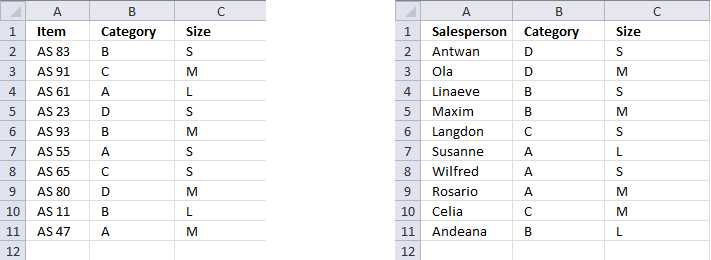This example demonstrates how to match multiple values from two different columns, both values must match.

Below is the merged list. The COUNTIFS function allows you to match multiple columns simultaneously.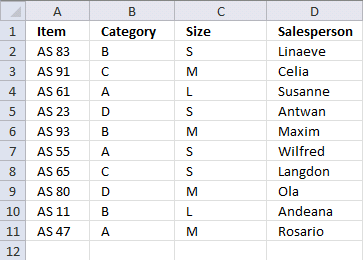Array formula in cell D2:

=INDEX('Ex 4 - Sheet2'!\$A\$2:\$A\$11, MATCH(1, COUNTIFS(B2, 'Ex 4 - Sheet2'!\$B\$2:\$B\$11, C2, 'Ex 4 - Sheet2'!\$C\$2:\$C\$11), 0))

This is an array formula and you need to enter this formula differently than a regular formula.

1. Type the formula in cell D2.
2. Press and hold CTRL + SHFT simultaneously.
3. Then press ENTER once.
4. Release all keys.

If you did it right, there are now curly brackets before and after the formula shown in the formula bar.

Copy cell D2 and paste it to D3:D11.

Note, you need to repeat the above steps if you edit the formula.

#### Explaining array formula in cell D2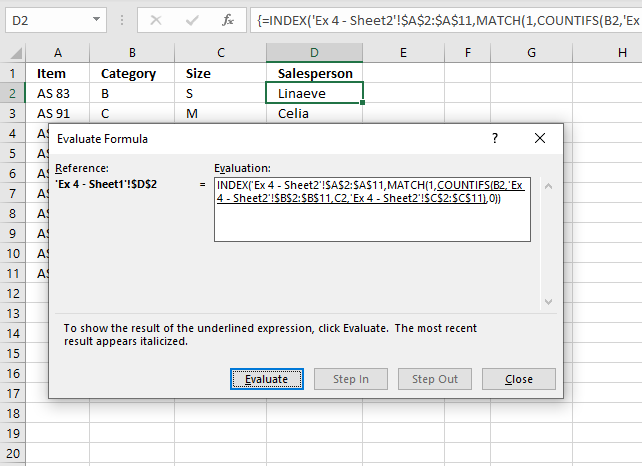The following link takes you to an explanation of how the "Evaluate Formula" tool works.

##### Step 1 - Check if value in cell B2 matches 'Ex 4 - Sheet2'!\$B\$2:\$B\$11 AND if value in cell C2 matches 'Ex 4 - Sheet2'!\$C\$2:\$C\$11

The COUNTIFS function allows you to use multiple arguments, in fact, up to 254 additional arguments.

It works just like the COUNTIF function meaning it counts the number of cells based on a condition, however, it also lets you use multiple criteria requiring you to enter the formula as an array formula.

COUNTIFS(criteria_range1criteria1, [criteria_range2criteria2]…)

We are going to use two conditions an compare them to two different columns, 1 equals True and 0 (zero) equals False. If both match on the same row the returned array returns 1 on the same relative position in the array.

COUNTIFS(B2,'Ex 4 - Sheet2'!\$B\$2:\$B\$11,C2,'Ex 4 - Sheet2'!\$C\$2:\$C\$11)

becomes

COUNTIFS("B",{"D"; "D"; "B"; "B"; "C"; "A"; "A"; "A"; "C"; "B"},"S",{"S"; "M"; "S"; "M"; "S"; "L"; "S"; "M"; "M"; "L"})

and returns {0; 0; 1; 0; 0; 0; 0; 0; 0; 0}

##### Step 2 - Find the relative position of 1

The MATCH function looks for 1 in the array and returns a number representing the relative position in the array. It returns an error value if not found at all.

MATCH(lookup_value, lookup_array, [match_type])

MATCH(1,COUNTIFS(B2,'Ex 4 - Sheet2'!\$B\$2:\$B\$11,C2,'Ex 4 - Sheet2'!\$C\$2:\$C\$11),0)

becomes

MATCH(1,{0; 0; 1; 0; 0; 0; 0; 0; 0; 0},0)

and returns 3 meaning number 1 is in position 3 in the array.

##### Step 3 - Return a value of the cell at the intersection of a particular row and column

The INDEX function then returns the value on the same row from cell range A2:A11 using the result from the MATCH function.

INDEX(array[row_num][column_num])

INDEX('Ex 4 - Sheet2'!\$A\$2:\$A\$11, MATCH(1, COUNTIFS(B2, 'Ex 4 - Sheet2'!\$B\$2:\$B\$11, C2, 'Ex 4 - Sheet2'!\$C\$2:\$C\$11), 0))

becomes

INDEX('Ex 4 - Sheet2'!\$A\$2:\$A\$11, 3)

and returns "Linaeve" in cell D2.